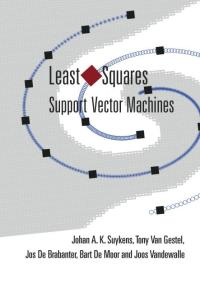# Least Squares SVM

Least Squares Support Vector Machines are a modification of the classical Support Vector Machine, please see Suykens et. al for a complete background.## LSSVM Regression¶

In case of LSSVM regression one solves (by applying the KKT conditions) the following constrained optimization problem.

\begin{align} & \min_{w,b,e} \ \mathcal{J}_P(w,e) = \frac{1}{2}w^\intercal w + \gamma \frac{1}{2} \sum_{k = 1}^{N} e^2_k \\ & y_k = w^\intercal \varphi(x) + b + e_k, \ k =1, \cdots, N \end{align}

Leading to a predictive model of the form.

\begin{equation} y(x) = \sum_{k = 1}^{N}\alpha_k K(x, x_k) + b \end{equation}

Where the values $\alpha \ \& \ b$ are the solution of

\begin{equation} \left[\begin{array}{c|c} 0 & 1^\intercal_v \\ \hline 1_v & K + \gamma^{-1} \mathit{I} \end{array}\right] \left[\begin{array}{c} b \\ \hline \alpha \end{array}\right] = \left[\begin{array}{c} 0 \\ \hline y \end{array}\right] \end{equation}

Here K is the $N \times N$ kernel matrix whose entries are given by $K_{kl} = \varphi(x_k)^\intercal\varphi(x_l), \ \ k,l = 1, \cdots, N$ and $I$ is the identity matrix of order $N$.

## LSSVM Classification¶

In case of LSSVM for binary classification one solves (by applying the KKT conditions) the following constrained optimization problem.

\begin{align} & \min_{w,b,e} \ \mathcal{J}_P(w,e) = \frac{1}{2}w^\intercal w + \gamma \frac{1}{2} \sum_{k = 1}^{N} e^2_k \\ & y_k[w^\intercal \varphi(x) + b] = 1 - e_k, \ k =1, \cdots, N \end{align}

Leading to a classifier of the form.

\begin{equation} y(x) = sign \left[ \sum_{k = 1}^{N}\alpha_k K(x, x_k) + b \right] \end{equation}

Where the values $\alpha \ \& \ b$ are the solution of

\begin{equation} \left[\begin{array}{c|c} 0 & y^\intercal \\ \hline y & \Omega + \gamma^{-1} \mathit{I} \end{array}\right] \left[\begin{array}{c} b \\ \hline \alpha \end{array}\right] = \left[\begin{array}{c} 0 \\ \hline 1_v \end{array}\right] \end{equation}

Here $\Omega$ is the $N \times N$ matrix whose entries are given by

\begin{align} \Omega_{kl} & = y_{k} y_{l} \varphi(x_k)^\intercal\varphi(x_l), \ \ k,l = 1, \cdots, N \\ & = y_{k} y_{l} K(x_k, x_l) \end{align}

and $I$ is the identity matrix of order $N$.

// Create the training data set

val data: Stream[(DenseVector[Double], Double)] = ...
val numPoints = data.length

// Create an implicit vector field for the creation of the stationary